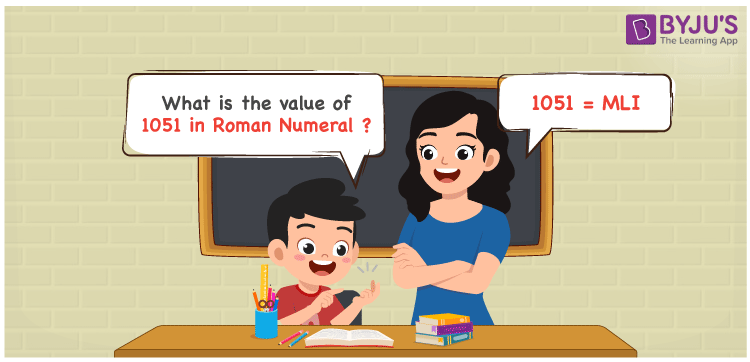# 1051 in Roman Numerals

1051 in Roman Numerals is MLI. You can convert 1051 to roman numerals considering the place value of each digit. Using the Roman numerals article, students will be strong with the conversion process and answer the questions related to this concept with ease. So, 1051 can be written in roman numerals as MLI.

 Number Roman Numeral 1051 MLI## How to Write 1051 in Roman Numerals?

In this section, the conversion of 1051 to roman numerals is explained in an interesting manner.

1051 = 1000 + 50 + 1

1051 = M + L + I

1051 = MLI

## Video Lesson on Roman Numerals## Frequently Asked Questions on 1051 in Roman Numerals

Q1

### Write 1051 in Roman Numerals.

1051 can be written in Roman Numerals as MLI.
Q2

### What is the value of 1000 + 51?

We know that
1000 in Roman Numerals = M
51 in Roman Numerals = LI
As 1000 + 51 = M + LI = MLI
So 1000 + 51 is 1051 which is written as MLI.
Q3

### How to write 1051 in Roman Numerals?

1051 can be converted to Roman Numerals by breaking the number according to its place value.
Thousands = 1000 = M
Tens = 50 = L
Ones = 1 = I
Number = 1000 + 50 + 1 = M + L + I = MLI